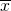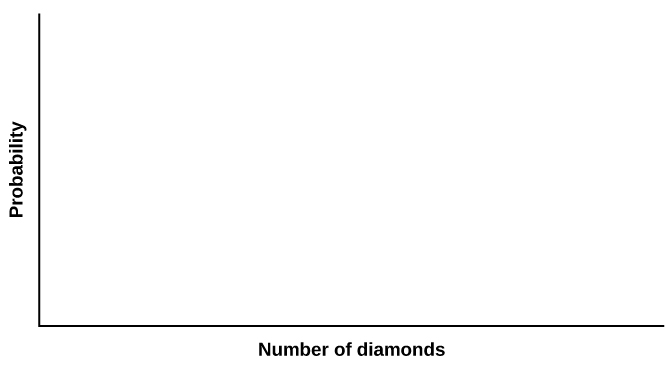Discrete Random Variables

# Discrete Distribution (Playing Card Experiment)

Discrete Distribution (Playing Card Experiment)

Class Time:

Names:

Student Learning Outcomes
• The student will compare empirical data and a theoretical distribution to determine if an everyday experiment fits a discrete distribution.
• The student will compare technology-generated simulation and a theoretical distribution.
• The student will demonstrate an understanding of long-term probabilities.
Supplies
• One full deck of playing cards
• One programming calculator

ProcedureThe experimental procedure for empirical data is to pick one card from a deck of shuffled cards.

1. The theoretical probability of picking a diamond from a deck is _________.
2. Shuffle a deck of cards.
3. Pick one card from it.
4. Record whether it was a diamond or not a diamond.
5. Put the card back and reshuffle.
6. Do this a total of ten times.
7. Record the number of diamonds picked.
8. Let X = number of diamonds. Theoretically, X ~ B(_____,_____)
Organize the Data
1. Record the number of diamonds picked for your class with playing cards in (Figure). Then calculate the relative frequency.
x Frequency Relative Frequency
0 __________ __________
1 __________ __________
2 __________ __________
3 __________ __________
4 __________ __________
5 __________ __________
6 __________ __________
7 __________ __________
8 __________ __________
9 __________ __________
10 __________ __________
2. Calculate the following:
1.= ________
2. s = ________
3. Construct a histogram of the empirical data.Theoretical Distribution
1. Build the theoretical PDF chart based on the distribution in the Procedure section.
x P(x)
0
1
2
3
4
5
6
7
8
9
10
2. Calculate the following:
1. μ = ____________
2. σ = ____________
3. Construct a histogram of the theoretical distribution.Using the Data

NOTE

RF = relative frequency

Use the table from the Theoretical Distribution section to calculate the following answers. Round your answers to four decimal places.

• P(x = 3) = _______________________
• P(1 < x < 4) = _______________________
• P(x ≥ 8) = _______________________

Use the data from the Organize the Data section to calculate the following answers. Round your answers to four decimal places.

• RF(x = 3) = _______________________
• RF(1 < x < 4) = _______________________
• RF(x ≥ 8) = _______________________

Discussion QuestionsFor questions 1 and 2, think about the shapes of the two graphs, the probabilities, the relative frequencies, the means, and the standard deviations.

1. Knowing that data vary, describe three similarities between the graphs and distributions of the theoretical, empirical, and simulation distributions. Use complete sentences.
2. Describe the three most significant differences between the graphs or distributions of the theoretical, empirical, and simulation distributions.
3. Using your answers from questions 1 and 2, does it appear that the two sets of data fit the theoretical distribution? In complete sentences, explain why or why not.
4. Suppose that the experiment had been repeated 500 times. Would you expect (Figure) or (Figure) to change, and how would it change? Why? Why wouldn’t the other table(s) change?